Resolving Seaborn plotting issue :

Being able to call sns.plt.show() is bad practice and the fact that it worked was a bug which the developer fixed. Unfortunately, there are many tutorials out there that still advise one to use sns.plt.show(). Here's a way to solve this problem :

• Import plt directly: import matplotlib.pyplot as plt
• Before plotting anything, set the default aesthetic parameters: sns.set() (important, because otherwise we won't get the Seaborn palettes).
• Replace all calls to sns.plt with plt.
In :
import pandas as pd
import numpy as np
import matplotlib.pyplot as plt
import seaborn as sns; sns.set()

# allow plots to appear in the notebook
%matplotlib inline


## Brain and body weight¶

This is a dataset of the average weight of the body and the brain for 62 mammal species. Let's read it into pandas and take a quick look:

In :
url = 'http://people.sc.fsu.edu/~jburkardt/datasets/regression/x01.txt'
col_names = ['id', 'brain', 'body']
mammals = pd.read_table(url, sep='\s+', skiprows=33, names=col_names, index_col='id')

Out:
brain body
id
1 3.385 44.5
2 0.480 15.5
3 1.350 8.1
4 465.000 423.0
5 36.330 119.5
In :
mammals.describe()

Out:
brain body
count 62.000000 62.000000
mean 198.789984 283.134194
std 899.158011 930.278942
min 0.005000 0.140000
25% 0.600000 4.250000
50% 3.342500 17.250000
75% 48.202500 166.000000
max 6654.000000 5712.000000

We're going to focus on a smaller subset in which the body weight is less than 200:

In :
# only keep rows in which the body weight is less than 200
mammals = mammals[mammals.body < 200]
mammals.shape

Out:
(51, 2)

We're now going to pretend that there are only 51 mammal species in existence. In other words, we are pretending that this is the entire dataset of brain and body weights for every known mammal species.

Let's create a scatterplot (using Seaborn) to visualize the relationship between brain and body weight:

In :
sns.lmplot(x='body', y='brain', data=mammals, ci=None, fit_reg=False)
plt.xlim(-10, 200)
plt.ylim(-10, 250)

Out:
(-10, 250)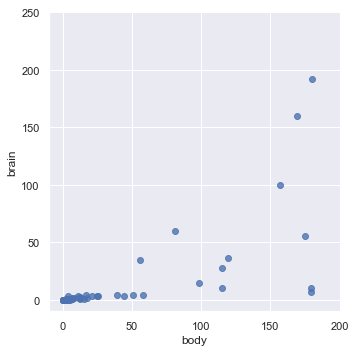There appears to be a relationship between brain and body weight for mammals.

## Making a prediction¶

Now let's pretend that a new mammal species is discovered. We measure the body weight of every member of this species that we can find, and calculate an average body weight of 100. We want to predict the average brain weight of this species (rather than measuring it directly). How might we do this?

In :
sns.lmplot(x='body', y='brain', data=mammals, ci=None)
plt.xlim(-10, 200)
plt.ylim(-10, 250)

Out:
(-10, 250)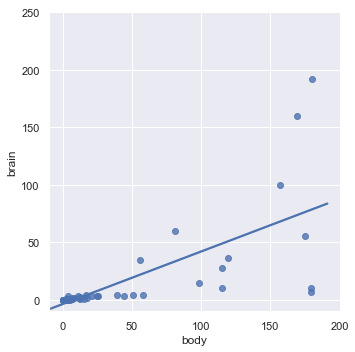We drew a straight line that appears to best capture the relationship between brain and body weight. So, we might predict that our new species has a brain weight of about 45, since that's the approximate y value when x=100.

This is known as a "linear model" or a "linear regression model", which we will study in a future class.

## Making a prediction from a sample¶

Earlier, I said that this dataset contained every known mammal species. That's very convenient, but in the real world, all you ever have is a sample of data. A more realistic situation would be to only have brain and body weights for (let's say) half of the 51 known mammals.

When that new mammal species (with a body weight of 100) is discovered, we still want to make an accurate prediction for the brain weight, but this task might be more difficult since we don't have all of the data that we would ideally like to have.

Let's simulate this situation by assigning each of the 51 observations to either universe 1 or universe 2:

In :
# set a random seed for reproducibility
np.random.seed(12345)

# randomly assign every observation to either universe 1 or universe 2
mammals['universe'] = np.random.randint(1, 3, len(mammals))

Out:
brain body universe
id
1 3.385 44.5 1
2 0.480 15.5 2
3 1.350 8.1 2
5 36.330 119.5 2
6 27.660 115.0 1

Important: We only live in one of the two universes. Both universes have 51 known mammal species, but each universe knows the brain and body weight for different species.

We can now tell Seaborn to create two plots, in which the left plot only uses the data from universe 1 and the right plot only uses the data from universe 2:

In :
# col='universe' subsets the data by universe and creates two separate plots
sns.lmplot(x='body', y='brain', data=mammals, ci=None, col='universe')
plt.xlim(-10, 200)
plt.ylim(-10, 250)

Out:
(-10, 250)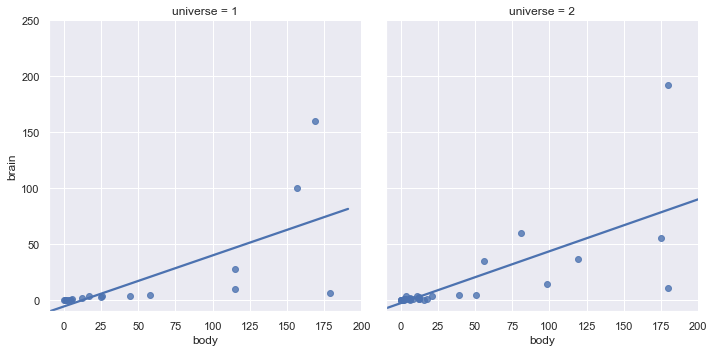The line looks pretty similar between the two plots, despite the fact that they used separate samples of data. In both cases, we would predict a brain weight of about 45.

It's easier to see the degree of similarity by placing them on the same plot:

In :
# hue='universe' subsets the data by universe and creates a single plot
sns.lmplot(x='body', y='brain', data=mammals, ci=None, hue='universe')
plt.xlim(-10, 200)
plt.ylim(-10, 250)

Out:
(-10, 250)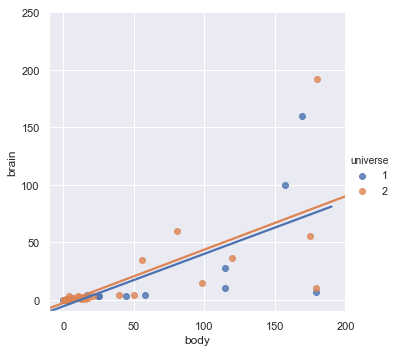What was the point of this exercise? This was a visual demonstration of a high bias, low variance model:

• It's high bias because it doesn't fit the data particularly well.
• It's low variance because it doesn't change much depending on which observations happen to be available in that universe.

## Let's try something completely different¶

What would a low bias, high variance model look like? Let's try polynomial regression, with an eighth order polynomial:

In :
sns.lmplot(x='body', y='brain', data=mammals, ci=None, col='universe', order=8)
plt.xlim(-10, 200)
plt.ylim(-10, 250)

Out:
(-10, 250)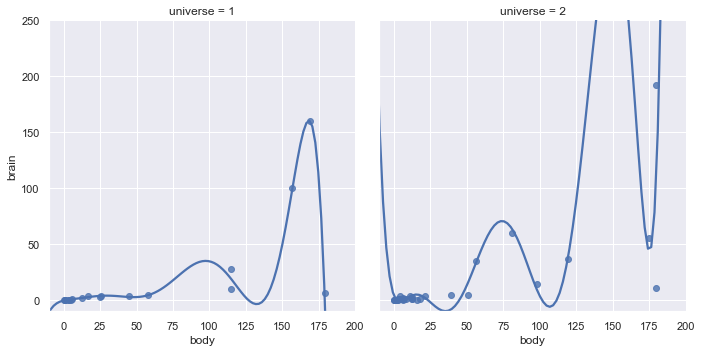• It's low bias because the models match the data quite well!
• It's high variance because the models are widely different depending on which observations happen to be available in that universe. (For a body weight of 100, the brain weight prediction would be 40 in one universe and 0 in the other universe!)

## Can we find a middle ground?¶

Perhaps we can create a model that has less bias than the linear model, and less variance than the eighth order polynomial?

Let's try a second order polynomial instead:

In :
sns.lmplot(x='body', y='brain', data=mammals, ci=None, col='universe', order=2)
plt.xlim(-10, 200)
plt.ylim(-10, 250)

Out:
(-10, 250)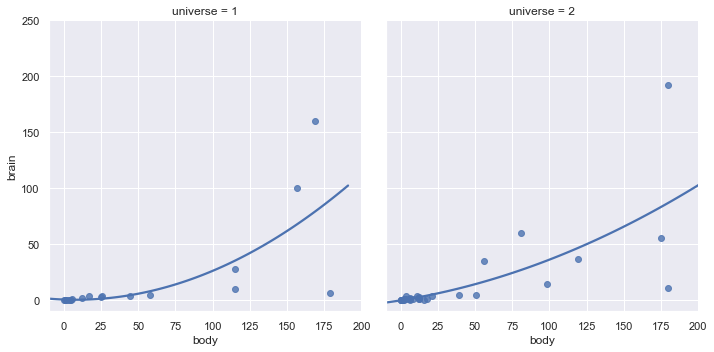This seems better. In both the left and right plots, it fits the data pretty well, but not too well.

This is the essence of the bias-variance tradeoff: You are seeking a model that appropriately balances bias and variance, and thus will generalize to new data (known as "out-of-sample" data).